Area of parallelogram

Chapter 9 Class 7 Perimeter and Area
Concept wise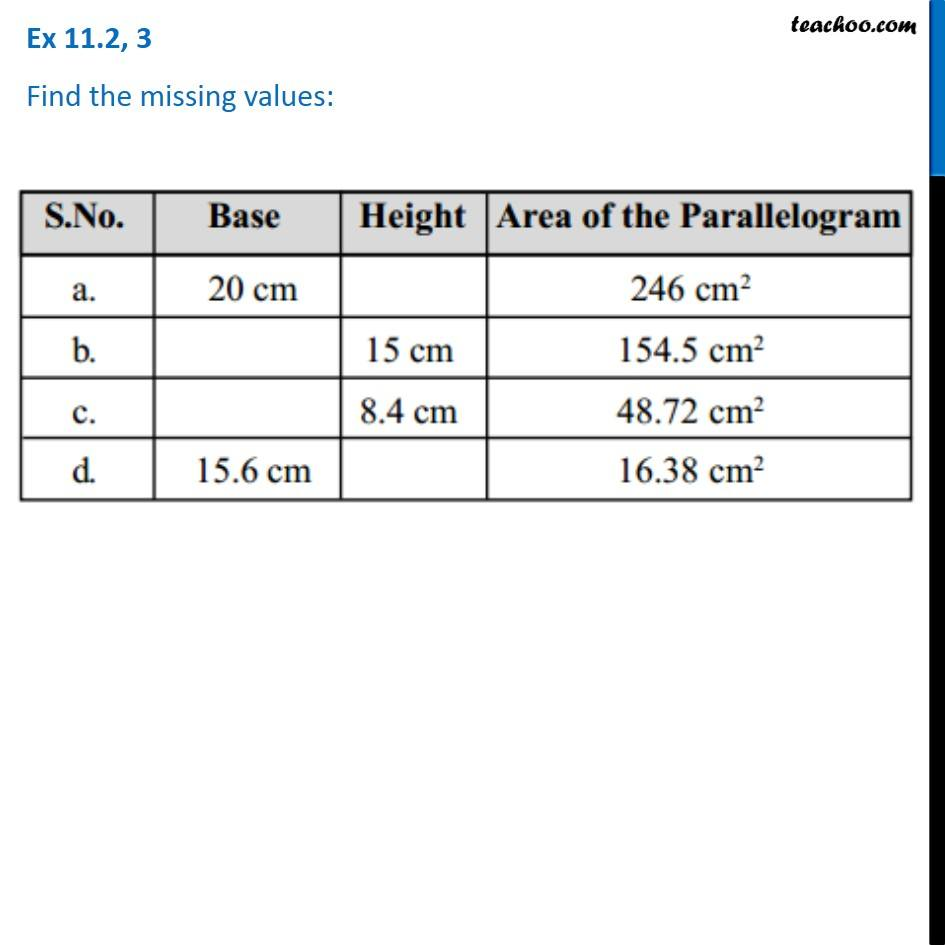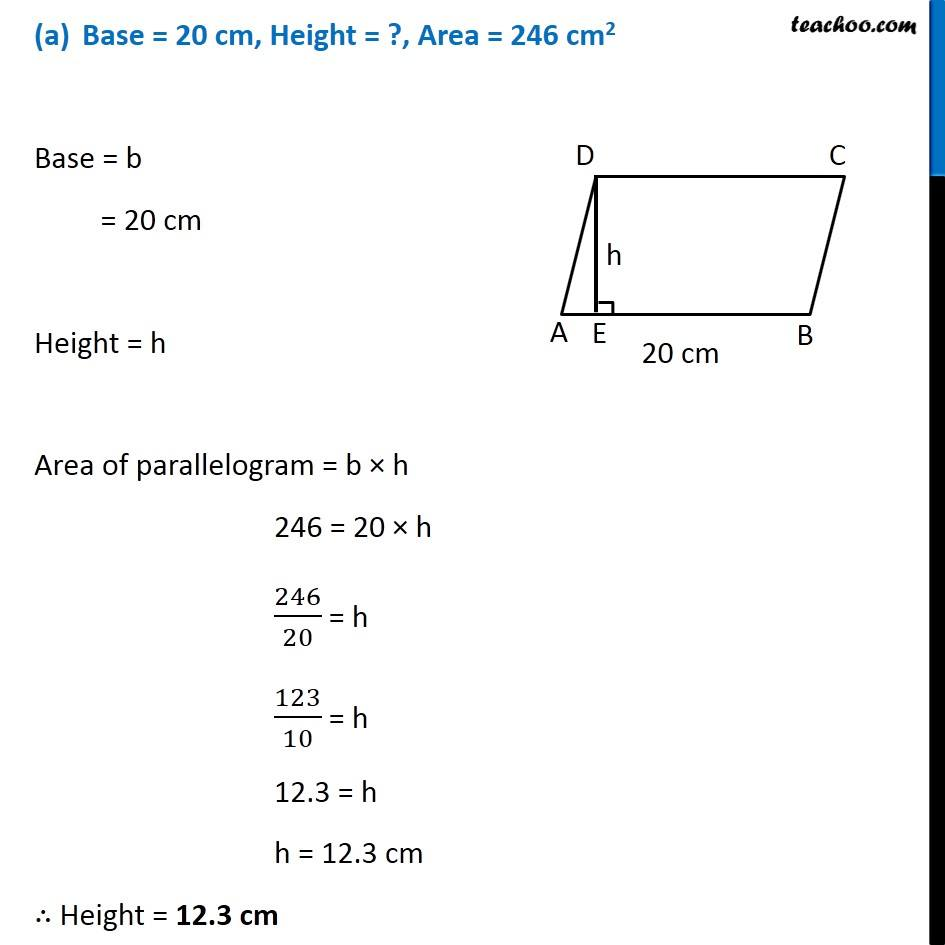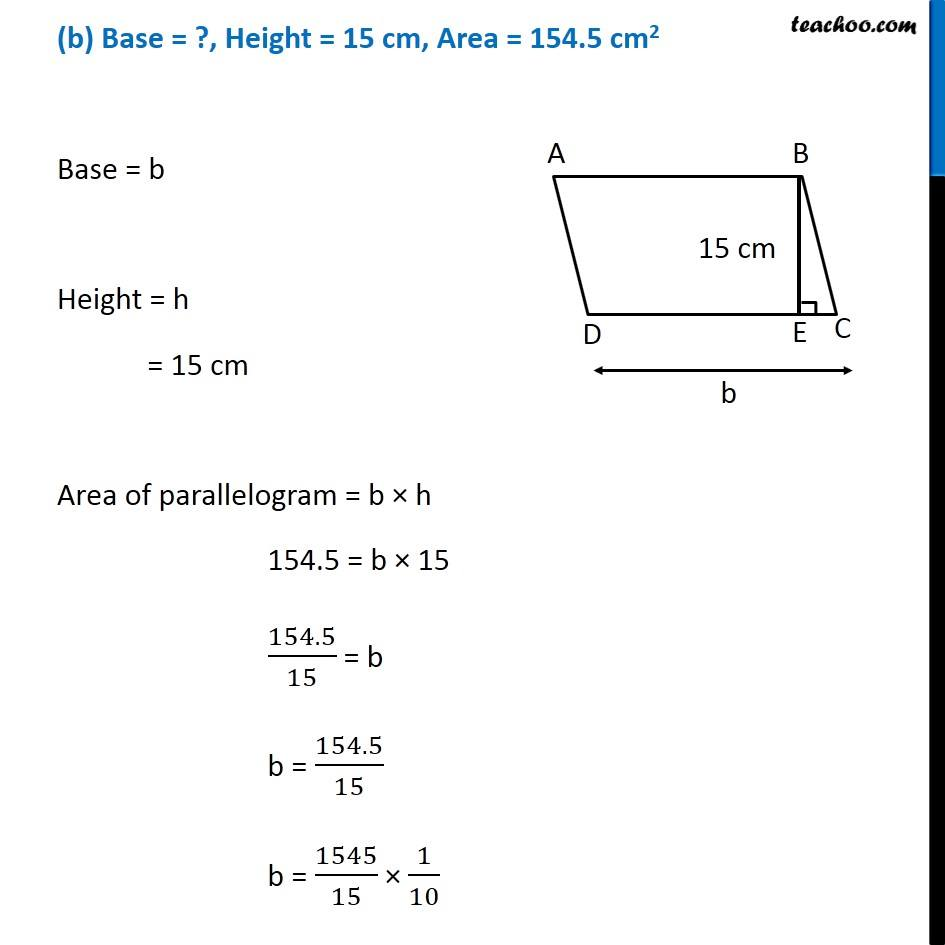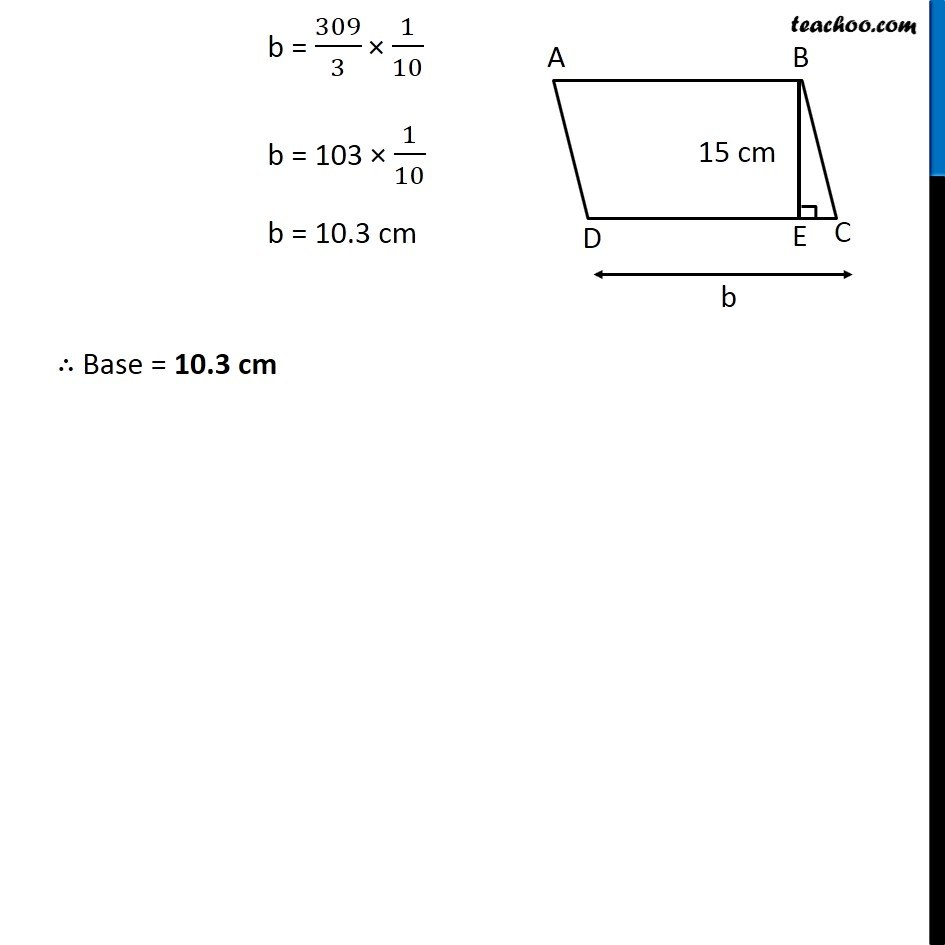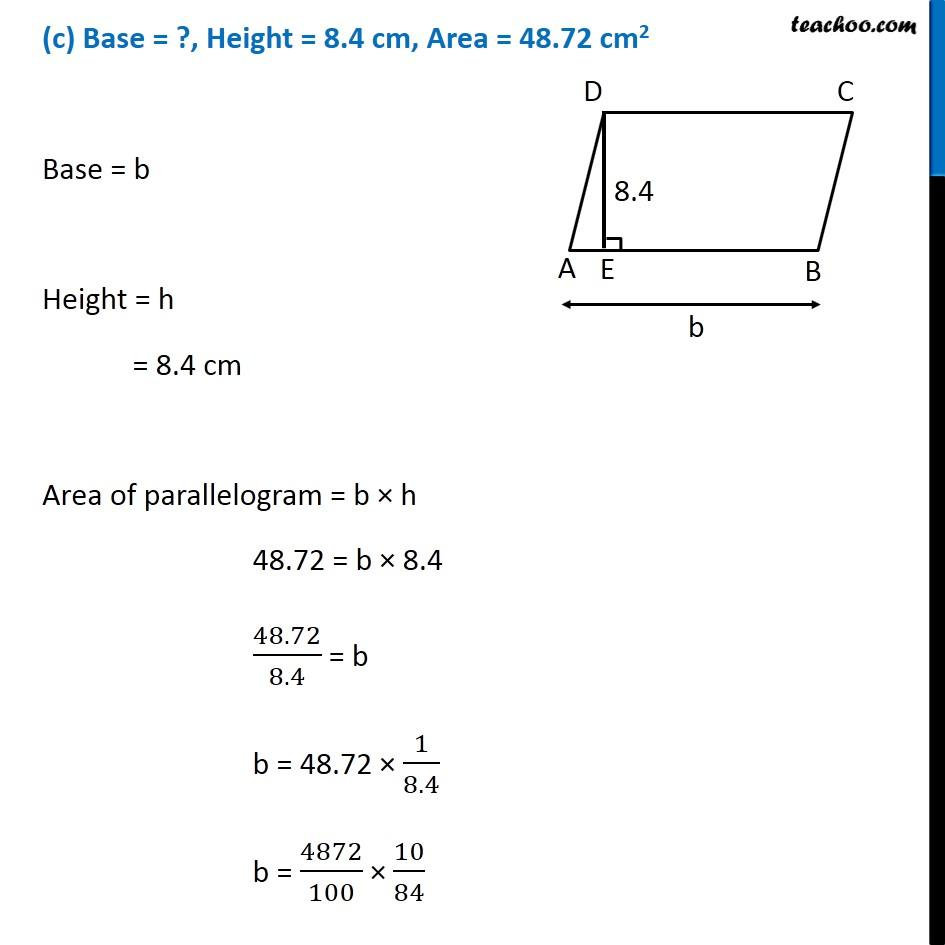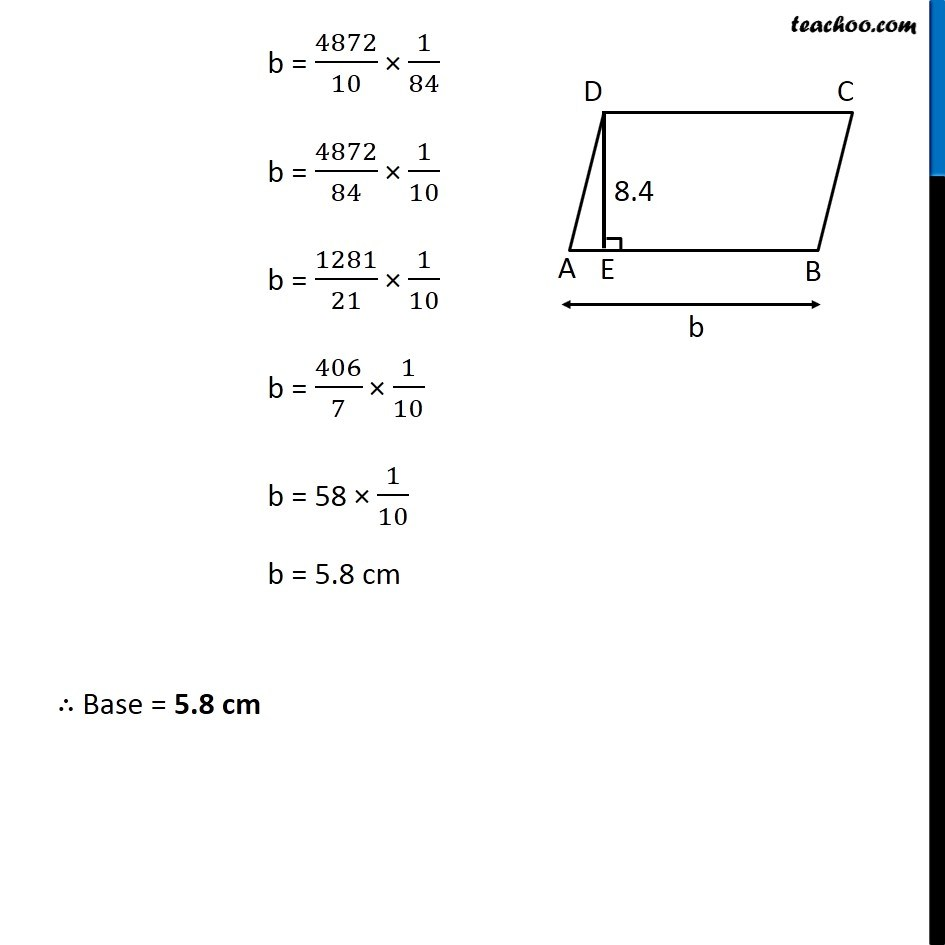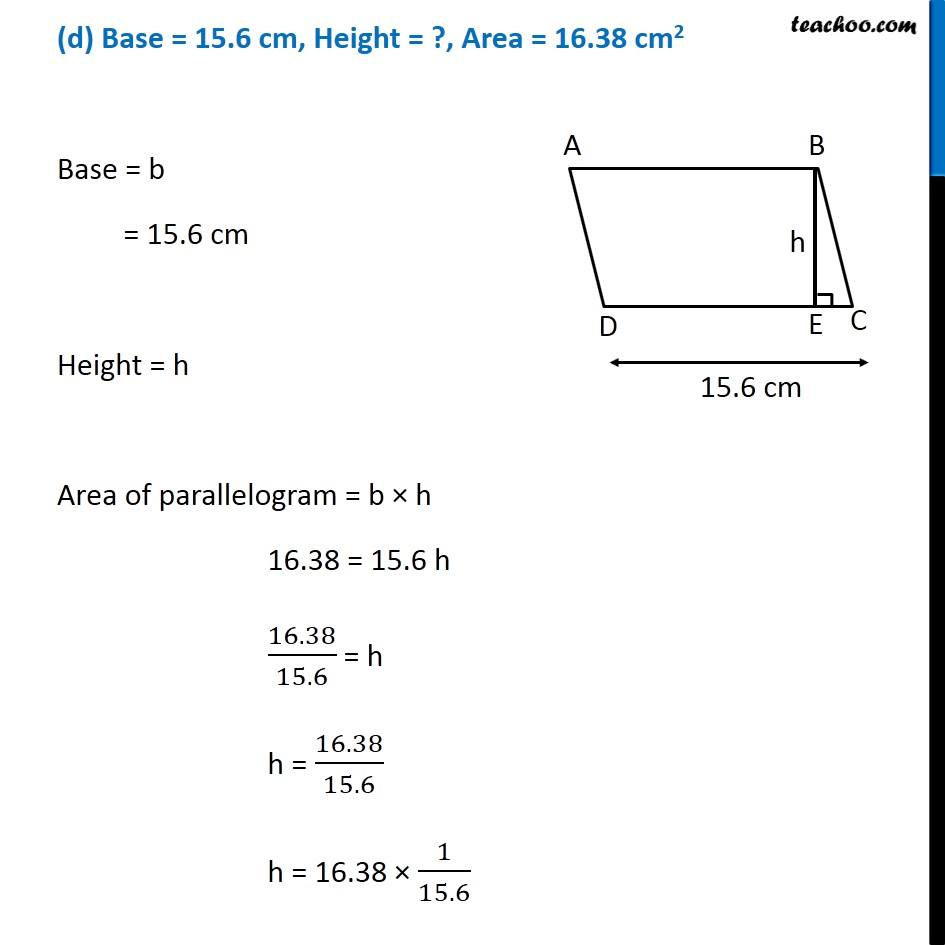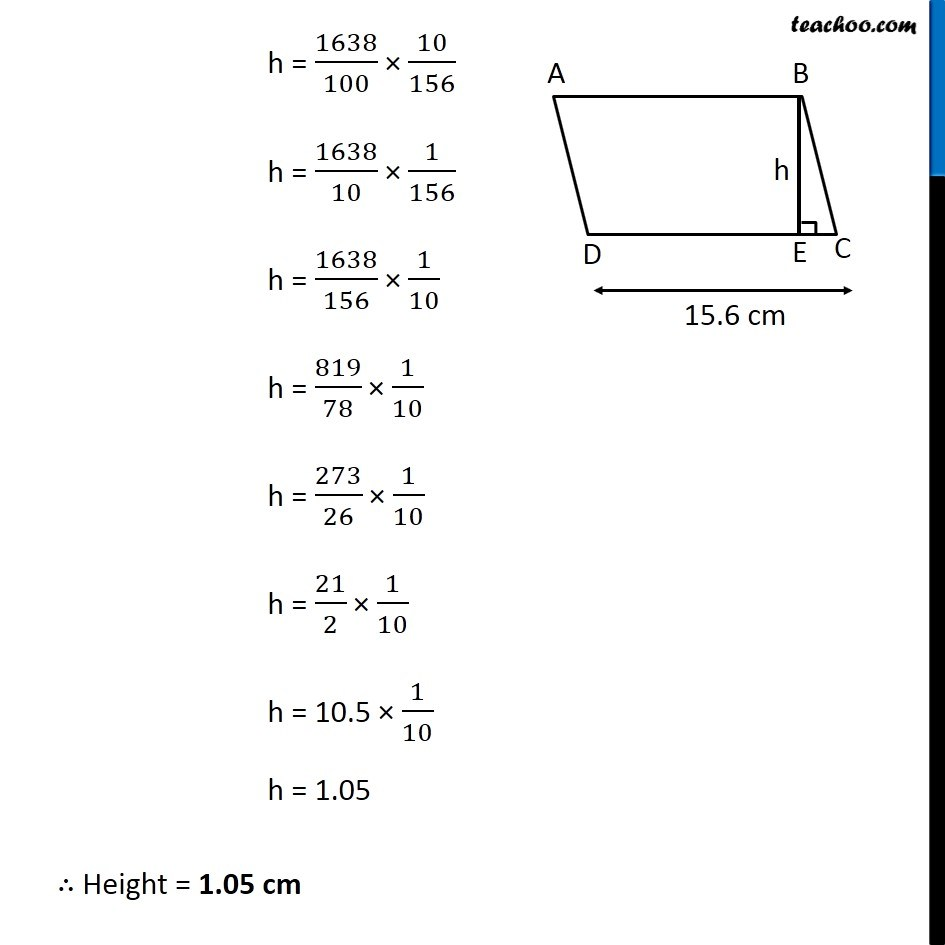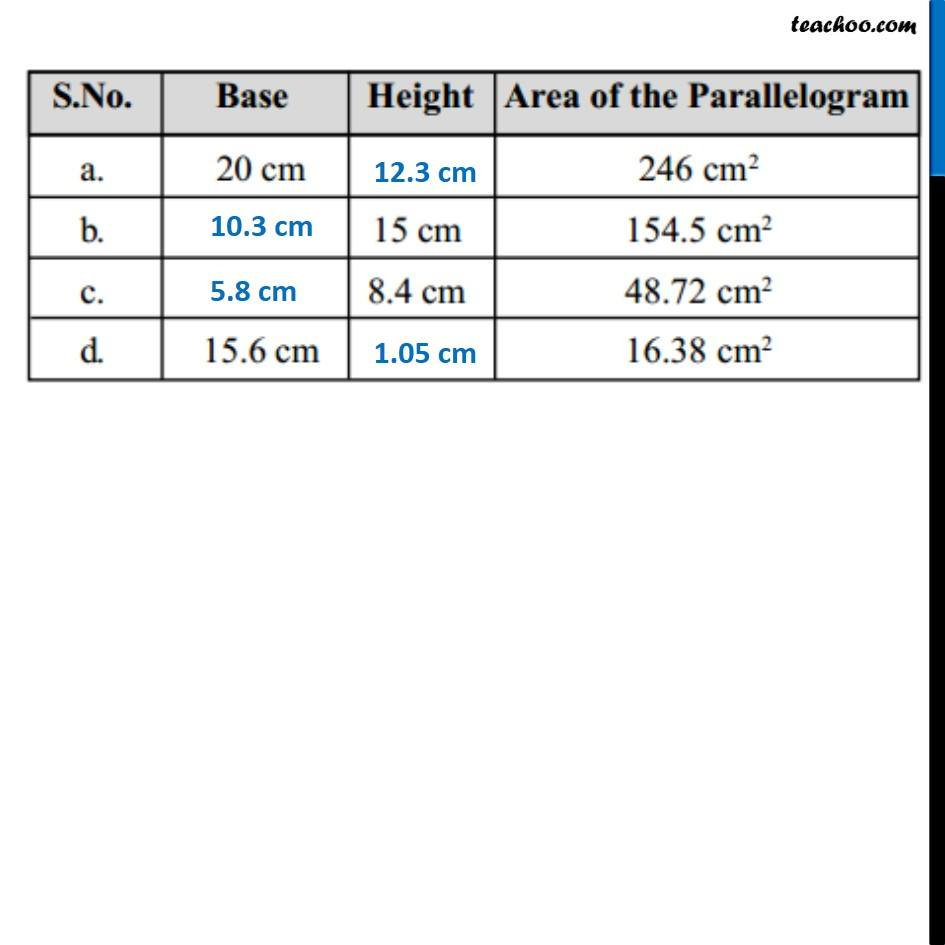Learn in your speed, with individual attention - Teachoo Maths 1-on-1 Class

### Transcript

Ex 9.1, 3 Find the missing values: Base = 20 cm, Height = ?, Area = 246 cm2 Base = b = 20 cm Height = h Area of parallelogram = b × h 246 = 20 × h 246/20 = h 123/10 = h 12.3 = h h = 12.3 cm ∴ Height = 12.3 cm (b) Base = ?, Height = 15 cm, Area = 154.5 cm2 Base = b Height = h = 15 cm Area of parallelogram = b × h 154.5 = b × 15 154.5/15 = b b = 154.5/15 b = 1545/15 × 1/10 b = 309/3 × 1/10 b = 103 × 1/10 b = 10.3 cm ∴ Base = 10.3 cm (c) Base = ?, Height = 8.4 cm, Area = 48.72 cm2 Base = b Height = h = 8.4 cm Area of parallelogram = b × h 48.72 = b × 8.4 48.72/8.4 = b b = 48.72 × 1/8.4 b = 4872/100 × 10/84 b = 4872/10 × 1/84 b = 4872/84 × 1/10 b = 1281/21 × 1/10 b = 406/7 × 1/10 b = 58 × 1/10 b = 5.8 cm ∴ Base = 5.8 cm (d) Base = 15.6 cm, Height = ?, Area = 16.38 cm2 Base = b = 15.6 cm Height = h Area of parallelogram = b × h 16.38 = 15.6 h 16.38/15.6 = h h = 16.38/15.6 h = 16.38 × 1/15.6 h = 1638/100 × 10/156 h = 1638/10 × 1/156 h = 1638/156 × 1/10 h = 819/78 × 1/10 h = 273/26 × 1/10 h = 21/2 × 1/10 h = 10.5 × 1/10 h = 1.05 ∴ Height = 1.05 cm